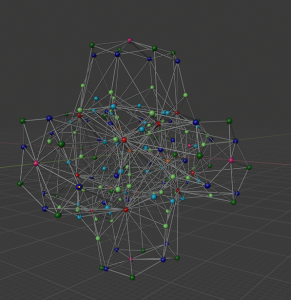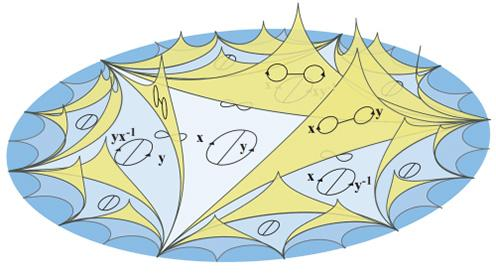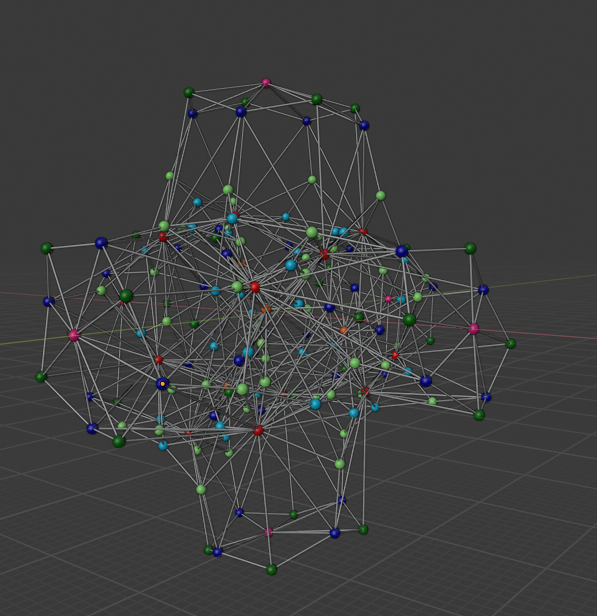# Outer space for the free group of rank 3

Date started: September 2019

###Abstract

Let$F_r$ be the free group of rank \$r\$. Introduced by Culler and Vogtmann he outer space of$F_r$ is a deformation spaces of graphs whose fundamental domain is isomorphic to$F_r$. It is the analogue of the Teichmüller space for surfaces. The outer space is defined as a simplicial complex. It is contractible and comes with the natural action of the outer automorphism group of the free group, which makes it a fundamental tool to study this group.

When$r = 2$, this space is very familiar. Basically it is a Farey tessellation of the hyperbolic plane with fans glued on it (see the first picture). When$r = 3$, the space is 5-dimensional. However its retracts onto a nice 3-dimensional simplicial complex called the spine of the outer space.

The goal of the project is to create pictures of the spine of the outer space for rank 3. It turns out that this space is highly complex.

The second picture show the 1-skeleton link of a particular vertex (the one associated to a wedge of 3 circles). It has over 150 faces.

### MediaThe outer space of F_2The 1-skeleton of the link of a particular vertex in the spine of the outer space of F_3. All the vertices with the same color are in the same orbit under the action of Out(F_3).

### References

• Culler, Marc and Vogtmann, Karen, Moduli of graphs and automorphisms of free groups, Inventiones Mathematicae (84) 91 – 119, 1986
• Bridson, Martin, Geodesic and curvature in metrical simplicial complexes, PhD. Thesis, 1991